PSoC™ 5, 3 & 1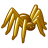Level 3
Level 3

# How much current does Charge Pump supply?

I try to use Charge Pump that can get in the Paper(AN60580)

but there is no spec of current.

so i ask to you, How much current does Charge Pump supply?

SIO sink current is 25mA, therefore maybe 25mA is maximum current.

is it true?

Thank you.

15 RepliesAnonymous
Not applicable

# Re: How much current does Charge Pump supply?

Hello there ! Thats an interesting question. Generally chargepumps are used to set reference voltages or used as level shifters and they are not used drive loads and hence it is not use case of charge pump to be used as current drive. As you keep drawing current from the load capacitor, the source(in our case SIO) should be capable of charging the capacitor back to maintian your required voltage. You could approximate the current capability of the charge pump built on the SIO to be about 12mA, since the current source(actual SIO pin)  is now available for only half the time. Actually its even lesser than 12mA.

Fret not !!! If you need additional current, you can connect more number of Pins in paralle.Level 3
Level 3

# Re: How much current does Charge Pump supply?

It is very helpful to me.

Thank you again.Anonymous
Not applicable

# Re: How much current does Charge Pump supply?

Good question,

and thanks for the answer U2.. Even I was curious about the same..Anonymous
Not applicable

# Re: How much current does Charge Pump supply?

thanks for the answer from  U2，

i know that  is 12ma  max  and generally  it  used as voltage reference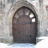Level 5Level 5

# Re: How much current does Charge Pump supply?

anyone have an example project with a charge pump with feedback -> comparator -> pwm?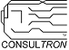Level 9Level 9

# Re: How much current does Charge Pump supply?

DaEr,

Maybe I can help.  However, I need some info from you.

• the voltage of VDDD?
• Your target output voltage of the charge pump?
• What is maximum load on the output voltage?  (This will determine the doubling caps.)  Note: the maximum of output source current for the SIOs is 4mA.  To get more than 4mA you need to parallel more outputs.

Len
"Engineering is an Art. The Art of Compromise."Level 5Level 5

# Re: How much current does Charge Pump supply?

• VDDD can ve either VBAT 2.0-3.0V or 1.8V fixed (via ldo from vbat)
• 3.0V fixed, i.e. so 50% up at 2.0V, vs more or less bypass when VBAT is >= 3.0V
• load will be less than 4mA, estimated 1-2mALevel 9Level 9

# Re: How much current does Charge Pump supply?

DaEr,

Thanks for the feedback.

I have good news for you.  In your case, you don't need the SIO in charge pump mode.

Infineon already provides a closed-loop feedback voltage boost regulator on-chip.

It's called the Boost Converter (Also known as the Inductive Boost Regulator)

Here's a link to the PSoC5LP datasheet. PSoC5LP datasheet

• 6.2.2   ( I suggest using configuration "Application of Boost Converter not powering PSoC device")
• 11
• 11.3.2

By setting the BOOST_CR0 register to the voltage you want to maintain, the regulator will SMP the VBAT voltage to the target voltage.

According to the datasheet, with the proper external values, you should be able to achieve 50mA if you needed.  Your target of 2mA shouldn't be a problem.

Len
"Engineering is an Art. The Art of Compromise."Level 5Level 5

# Re: How much current does Charge Pump supply?

Much appreciated Len!

Actually running a PSoC 6, so will try find a way there. Also a bit short on pins, so the goal is to do this with just two, switching the direction of the lower fet to allow reading back the feedback without the need for a 3rd pinLevel 9Level 9

# Re: How much current does Charge Pump supply?

DaEr,

You're welcome.

I don't think the PSoC6 has SIOs.  However, for the SMPS operation, I think any digital should work as long as one output can be configured as open drain high and the other as push-pull and the diodes are in place.

Len
"Engineering is an Art. The Art of Compromise."Level 9Level 9

# Re: How much current does Charge Pump supply?

DaEr,

I've LTSpice simulated the open-loop (original) charge pump circuit with equivalent components.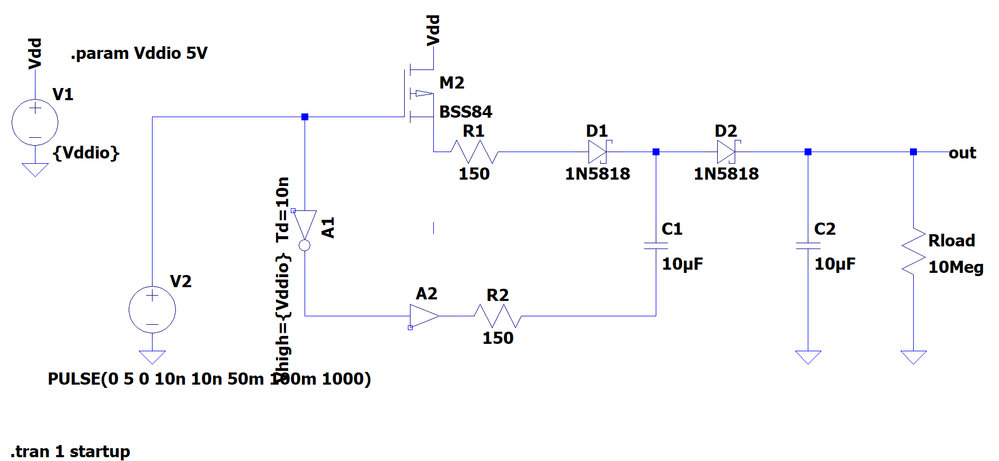Note: The 150 ohm resistors (R1 and R2) are to approximate the source resistance of the drivers.  A2 is a push-pull buffer and M2 is the open drain FET.

Here is the simulated results.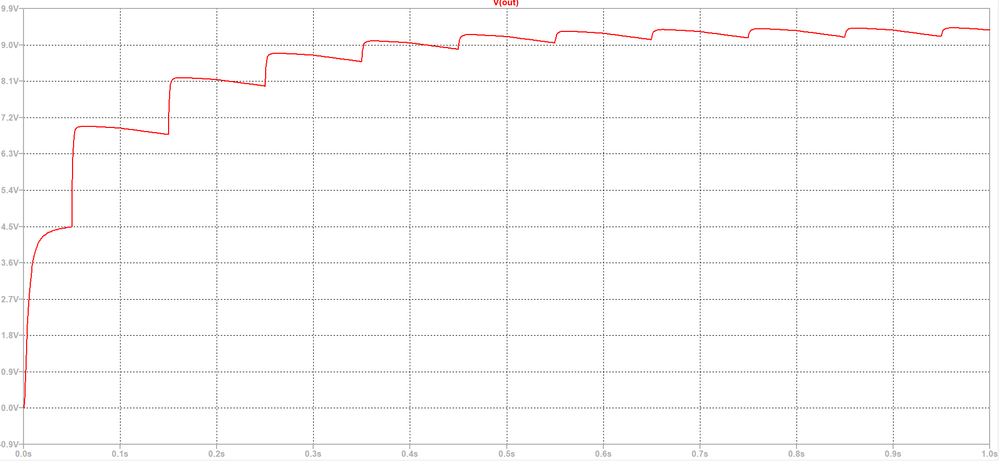The goal of the charge pump circuit is to have an output 'near' 2 * Vddio.  The reality, it will always be less than this value.

Here are some design considerations:

• The diodes preventing reverse current flow have a voltage drop.  Each diode forward voltage drop will subtract from 2 * Vddio.  Therefore if using standard silicon diodes with a forward voltage of 0.6V, then the output will be closer to Vout = (2*Vddio) - (2 * 0.6V).   Using a schottky diode as shown in my circuit, the forward voltage drop is about 0.2V.  In this case Vout = (2*Vddio) - (2 * 0.2V)
• If using the USB to power the PSoC, the nominal voltage from USB is < 5.0V.  For example the USB VDD interface has a single Schottky diode (Vfd = 0.2V).  Therefore 'if' the USB host is outputting 5.0V, then the PSoC Vddio = 5.0V -0.2V = 4.8V.   Usually I measure about 4.7V in many cases.
• The two external storage caps are 10uF each.  A smaller value will have more voltage bounce.  A higher value less bounce.  (Note:  In this circuit simulation, the load is >10Meg.
• A load on the output of more than 100K ohms is probably less than useful especially in a closed-loop charge pump circuit.  Below is plot of Vout with Rload = 100K ohm.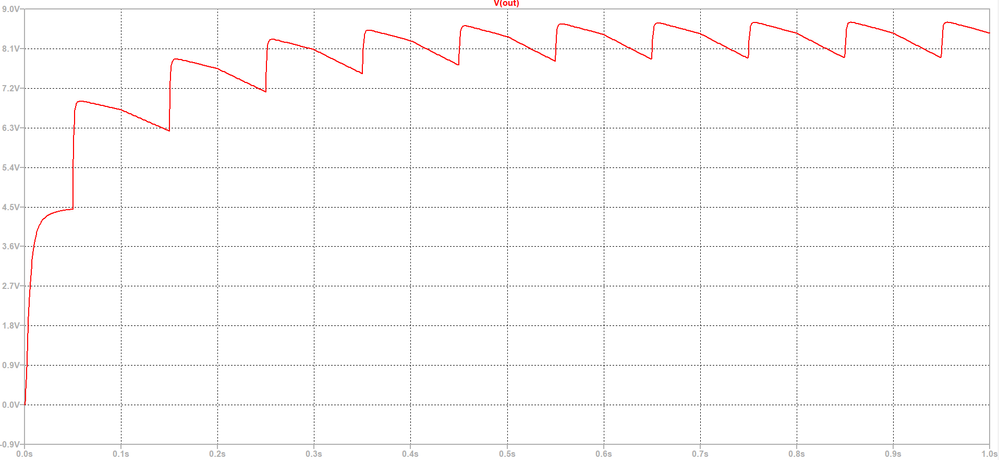• If you want a more stable Vout, you need higher values of caps.  The downside is that it will take longer to stabilize Vout due to the source resistance of the drivers contributing to the RC factor of charging the caps.
• A way to increase the Vout stability is to increase the switch-mode clock frequency from 10KHz.   I when to 100KHz and the caps were more effective.  Below is a plot with the clock at 100KHz.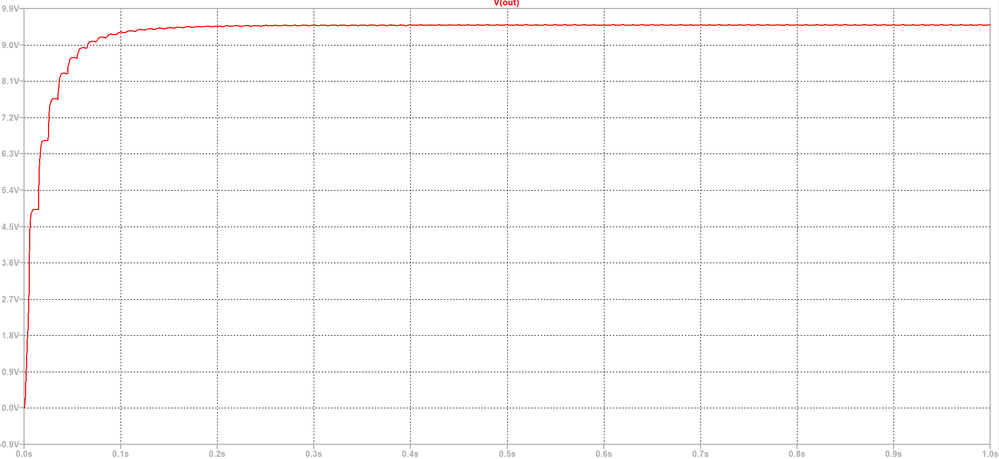The downside of this design choice is that the EMI generated by the higher clock and larger voltages and currents might be an issue in commercial designs.

I'm working on a closed-loop simulation with a comparator and a gated switch-mode clock.

Len
"Engineering is an Art. The Art of Compromise."Level 9Level 9

# Re: How much current does Charge Pump supply?

DaEr,

I have an untested Charge pump closed loop circuit.

Here is my TopDesign of it: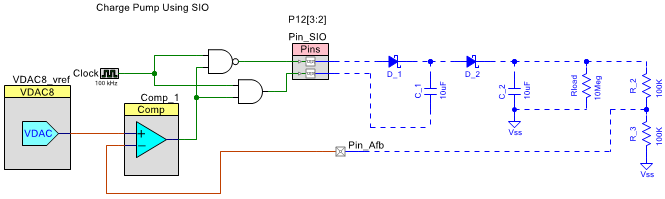TopDesign of the Charge Pump Closed Loop

Here are some design considerations:

• The circuit can regulate from 4.5V to about 7.6V.  The lower limit is due to some current flow through the open drain high pin.   The upper limit is due to the diode drops and the switch-mode switching factor and that VDAC_vref upper voltage is 4.080V.
• Set the VDAC_vref value = Target Voltage/2.  With VDAC_vref value = 4V, then the Target Voltage is (4.0V * 2 = 8.0V)- the 2 diode voltage drops (2*0.2V = 0.4V) = 7.6V.
• If you assume a 0.4V diode drop, you can compensate for it by adjusting VDAC_vref by addiing 0.4V/2 to the value.
• The higher the load, the lower the maximum regulated charge pump voltage.

Here's the LTSpice sim of this circuit: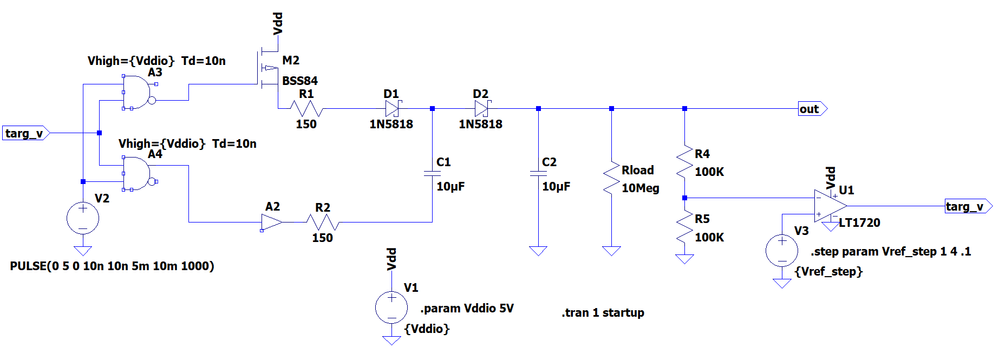Charge Pump Closed Loop Sim Circuit

Here are two Vout plots.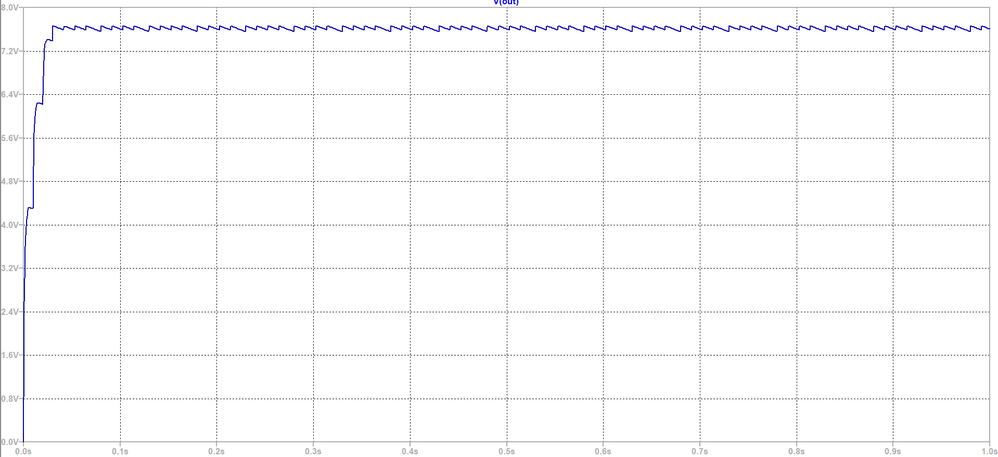Vout with VDAC_vref = 4.0V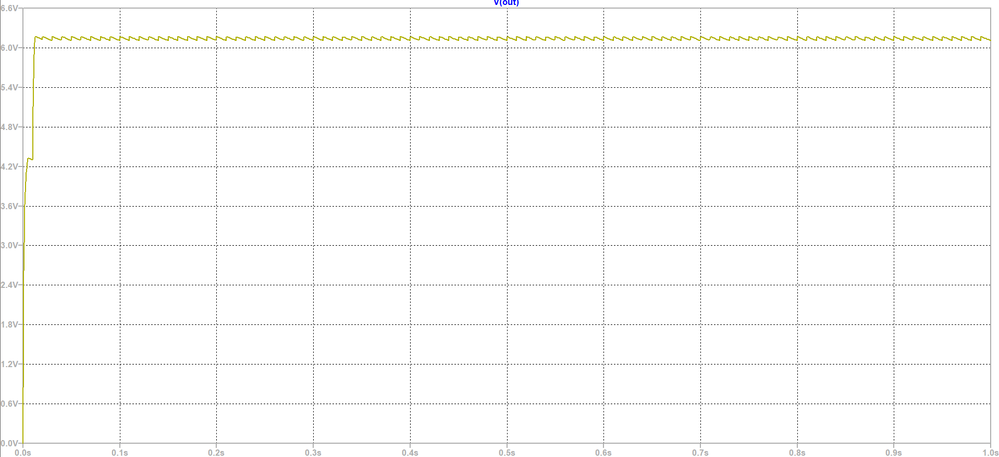Vout with VDAC_vref = 3.2V

If you are looking to regulate the output voltage below Vddio, there are two better ways to do this.

• You set the SIO output to be Vref'd (Using the VDAC8).  Refer to the App Note your referenced section 3.2.  The maximum current is about 4mA per pin.  You can parallel the pins for more current.
• The other way is to use the VDAC8 through an opamp.  Using specific PSoC pins designed to have low output resistance to an OpAmp output (pins P0.0, P0.1, P3.6, P3.7).  You can achieve nearer 25mA.  I believe this is the best method.
Len
"Engineering is an Art. The Art of Compromise."Level 5Level 5

# Re: How much current does Charge Pump supply?

Hi again Len, thanks for such an in depth reply.

I'm trying to come up with a way to reduce the solution from 3 pins to 2 pins.
The output of the charge-pump will actually feed one IO-bank of the psoc, so perhaps there are some ways to do internal analog routing of a VDDIO bank rail to the comparator?

Option 2 would be to dynamically switch the Pin tied to C1 to an input -> sampleLevel 9Level 9

# Re: How much current does Charge Pump supply?

DaEr,

If you're planning on using SIO pins your solution is:

• 2 pins without voltage feedback

If you're using GPIO pins is is the same as the SIO pins.

Have you considered the Boost Converter solution?  It is a 3 pin solution a well but it uses dedicated pins that cannot be doubled as GPIO.  (VBAT,IND,VBOOST)

Option 2:  Since there is a diode isolating the pin providing the + positive to C1, you can't read a useful feedback voltage.

Len
"Engineering is an Art. The Art of Compromise."Level 5Level 5

# Re: How much current does Charge Pump supply?

we'll build a prototype and test this using "OVT" pins (SIO not avail on psoc 6)

managed to find an extra gpio to measure the output, so let's see!